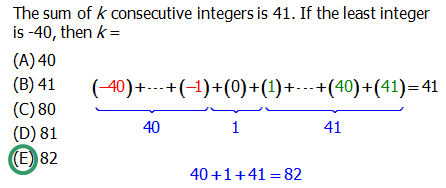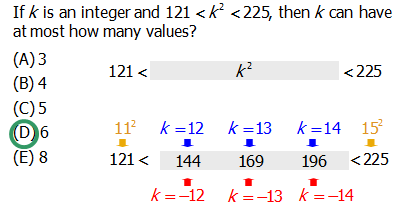# Inclusive Counting

Question #1: On Monday, there were 29 bananas in the cafeteria. No new bananas were brought in after Monday. Two days later, on Wednesday, there were 14 bananas left. How many were eaten in that time?

Question #2: In January of last year, MicroCorp start-up had 14 employees. All of those employees have stayed on through June. In June of last year, they had 29 employees. How many new employees did they hire?

Clearly for both of these questions, we need simply the different: 29 - 14 = 15. That’s the correct answer for each of them. Now, try a very different kind of question, with a different answer.

Question #3: A certain workshop begins on the 14th of this month and ends on the 29th of this month. How many days long is this workshop?

Question #4: How many multiples of 5 are there from 70 to 145?

Those two have the same answer as each other, but it’s not the same as the answer for #1 and #2.

## Inclusive

We say the final two require “inclusive” counting, because both endpoints are included, whereas in the first two questions, both endpoints were not included. What do I mean?

In Question #1, the 29th banana was eaten, the 28 banana was eaten, …. the 15 banana was eaten, BUT the 14th banana was not eaten because there 14 remaining. The endpoint 14 is “not included” in those eaten.

In Question #2, employees #1 - #14 were already hired by last January. Employees #15 - #29 were new hires. The lower endpoint, employee #14, was “not included” in the group of new hires.

Now, by contrast, the 14th is the first day of the workshop, and the 29th is the last day of the workshop. Both the 14th and 29th are days when the workshop is happening. Both endpoints are included.

Similarly, in #4, the multiples of 5 from 70 to 145 include both 70 and 145. Again, both endpoints are included. Incidentally, the connection to the other three questions: 70 = 5*14 and 145 = 5*29, so the list of multiples of 5 from 70 to 145 is really 5 times the list of consecutive integers from 14 to 29, so the underlying question is really: how many consecutive integers are there from 14 to 29?

As may be apparent, the inclusive scenario always includes exactly one more member than the “not included” scenario. Therefore, the formula for inclusive counting is

number \space included = (last) - (first) + 1

Notice, this only works if the numbers are consecutive. If the question is like #4, you may have to notice what change you can make to match the given list with a list of consecutive integers.

## Practice questions

Q1. The sum of k consecutive integers is 41. If the least integer is -40, then k =

A. 40

B. 41

C. 80

D. 81

E. 82Q2. If k is an integer and 121 \lt k^2 \lt 225, then k can have at most how many values?

A. 3

B. 4

C. 5

D. 6

E. 8FAQ: How would I know to consider the negative values too? A: You should always make it a point to consider negative values when you're looking for the values of a number that is being squared (^2) or is being multiplied to the 4th power (^4), etc. Basically, any even exponent (^2, ^4, ^6, ^8, etc.) means that's there's a possibility that the value of the base/your answer may be negative.